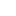lang="en-US"> [100% off] Machine Learning - Regression and Classification (math Inc.) | MY VIP TUTO

# [100% off] Machine Learning – Regression and Classification (math Inc.)

Enrol for this paid Udemy course Machine Learning – Regression and Classification (math Inc.) now for free using the 100% discount link we’ve provided below.

This is a complete Beginner to Advance level guide to Machine Learning. Hands-on Learning approach with in-depth math concepts.

## What you’ll learn

• Understand and implement a Decision Tree in Python
• Understand about Gini and Information Gain algorithm
• Solve mathematical numerical related decision trees
• Learn about simple, multiple, polynomial and multivariate regression
• Learn about Ordinary Least Squares Algorithms
• Solve numerically related to Ordinary Least Squares algorithm
• Learn to create real-world predictions and classification projects
• Learn about Logistic Regression and hyperparameters

## Machine Learning – Regression and Classification (math Inc.) – Course Description

Machine learning is a branch of artificial intelligence (AI) focused on building applications that learn from data and improve their accuracy over time without being programmed to do so.

In data science, an algorithm is a sequence of statistical processing steps. In machine learning, algorithms are ‘trained’ to find patterns and features in massive amounts of data in order to make decisions and predictions based on new data. The better the algorithm, the more accurate the decisions and predictions will become as it processes more data.

Machine learning has led to some amazing results, like being able to analyze medical images and predict diseases on-par with human experts.

Google’s AlphaGo program was able to beat a world champion in the strategy game go using deep reinforcement learning.

Machine learning is even being used to program self driving cars, which is going to change the automotive industry forever. Imagine a world with drastically reduced car accidents, simply by removing the element of human error.

Topics covered in this course:

1. Lecture on Information Gain and GINI impurity [decision trees]

2. Numerical problem related to Decision Tree will be solved in tutorial sessions

3. Implementing Decision Tree Classifier in workshop session [coding]

### Recommended Anime to watch this 2022### Recommended App for StudentsDeveloper: 237 SOLUTIONS
Price: Free

4. Regression Trees

5. Implement Decision Tree Regressor

6. Simple Linear Regression

7. Tutorial on cost function and numerical implementing Ordinary Least Squares Algorithm

8. Multiple Linear Regression

9. Polynomial Linear Regression

10. Implement Simple, Multiple, Polynomial Linear Regression [[coding session]]

11. Write code of Multivariate Linear Regression from Scratch

## Recommended to store and share your imagesDeveloper: 237 SOLUTIONS
Price: Free

13. Lecture on Logistic Regression [[decision boundary, cost function, gradient descent…..]]

14. Implement Logistic Regression [[coding session]]

## Machine Learning – Regression and Classification (math Inc.) Coupon

More Udemy course coupons here